# Numerical analysis of natural convection in a triangular cavity with different configurations of hot wall

Numerical analysis of natural convection in a triangular cavity with different configurations of hot wall

Manoj K. TriveniRajsekhar Panua

Department of Mechanical Engg, National Institute of Technology, Agartala, Tripura, 799046, India

Corresponding Author Email:
triveni_mikky@yahoo.com
Page:
11-18
|
DOI:
https://doi.org/10.18280/ijht.350102
|
Accepted:
|
Published:
31 March 2017
| Citation

OPEN ACCESS

Abstract:

This computational work is exploited in an isosceles right triangular cavity to delineate the effect of different shapes of base hot wall i.e. smooth, triangular zig-zag (TZ) and caterpillar shape (CS) on natural convection cooling. The side and inclined wall of the enclosure is presumed as cold walls. The two dimensional mass, momentum and energy equations are solved by finite volume method based on SIMPLE algorithm. The results are drawn for different types of hot wall with varying aspect ratio (σ) and Rayleigh number (105-107). From the investigation, it is found that the heat transfer rate is increased for both triangular zig-zag and caterpillar curve shape walls and with the increase of their aspect ratios but the maximum rate of heat transfer is obtained for caterpillar shape.

Keywords:

Hot Wall Configurations, Triangular Cavity, Natural Convection, Rayleigh Number

1. Introduction

The heat transfer process associated with natural convection is extensively involved in numerous engineering applications due to its diverse applications in geophysics, nuclear reactor system, energy efficient buildings, cooling of electronics system, solar system etc. Ample literatures are available on various geometrical shaped enclosures such as square, rectangular, trapezoidal, quadrantal etc. heated or cooled from the enclosure walls [1-7]. Heat transfer analysis in triangular enclosures heating from side or below are substantially employed in various domestics and industrial applications [8–11]. Varol et al.  have numerically investigated the natural convection in triangular enclosure with heater. It was reported that the aspect ratio and height of the heater shall be higher and heater must be placed at the center of the bottom wall for high heat transfer. Mahmudi et al.  studied the natural convection in a square cavity filled with nanofluid. It was found that the heat transfer was linearly increased with the increase of nanoparticles concentration. Triveni et al.  performed a numerical work in a right triangular enclosure for partially heating and cooling. They found that the heat transfer rate was more pronounced at the Left-Bottom (LB) position compared to other positions. Triveni et al.  have again investigated the natural cooling of partial hot wall through different configurations of cold walls. It was concluded that the the higher heat transfer rate was found for the cold wall configuration AB when the partial hot wall was placed at the left side on the base wall. Bouabdallah et al.  investigated the natural convection in a rectangular cavity.  They reported that the average Nusselt number was increased with the increase of Rayleigh number. There are plenty of studies informed that the heat transfer rate is augmented for irregular surface than the plane surface. Dalal et al.  examined the natural convection in a square cavity with the wavy wall on the right side. Numerical results explored that the average Nusselt number was increased on wavy wall at low Rayleigh number while decreased with the increase of Rayleigh number. Bhardwaj et al.  studied the heat transfer and entropy generation in right triangular cavity with wavy wall. It was observed that the undulation on the left wall was increased the Nusselt number by 53%, at Da = 10-2 and Ra = 106 as compared to the no-undulation case. Rahman et al.  studied the heat transfer in a corrugated triangular enclosure. Results revealed that the both heat and mass transfer were enhanced with the increase of the wave length and Rayleigh number. Hatmi and Safari  studied the free convection in wavy enclosure filled with nanofluid with a heated cylinder located inside. They found that the heat transfer was higher when the cylinder was located at the center. Cho  numerically examined the naturl convection in wavy enclosure filled with nanofluid. Results reported that the Nusselt number was decreased with the increase of wave amplitude and increased with Rayleigh number. Sheremet et al.  numerically investigated the natural convection in a porous wavy enclosure filled with nanofluid. They stated that heat transfer was enhanced with the increment of Rayleigh number and decrease of undulation number. Shirvan et al.  investigated the natural convection in a wavy square cavity filled with nanofluid. It was observed that the heat transfer rate was increased for wave amplitude and wavelengths at fixed Rayleigh number. However, Nusselt number was increased with the increase of volume fraction. Sheremet et al.  investigated the natural convection in a wavy triangular cavity. It was reported that the average Nusselt number was decreased with the increase of undulation number.

The brief literature survey provides evidence that studies on enclosures with the corrugated structure of the wall plays significant role in the heat transfer enhancement. The irregular shapes have many practical applications in flat-plate solar collectors, refrigeration systems, and microelectronic devices [19, 25]. Triangular enclosures with irregular wall have received very little attention however right triangular cavity with corrugated wall as shown in Fig. 1 has not been explored so far. Thus, the main objective of this study is to examine the effect of different geometrical configuration of the base wall of a right triangular cavity on heat transfer rate.

2. Description of The Physical Model and Mathematical Formulation

## 1.png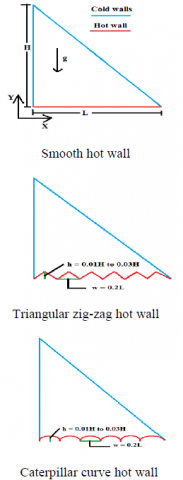Figure 1. Schematic diagram of the physical models of triangular cavity

A physical model of isosceles right triangular cavity of length L and height H has been drawn with the coordinate system for the present study and presented in figure 1. The base wall of the enclosure is considered as hot wall and maintained at constant temperature Th. The side and inclined walls are maintained at temperature Tc which lowers than the temperature of base wall. The shape of the base wall is altered from one smooth to triangular and caterpillar curve shape with different heights. The width of the shape (w) is fixed at 0.2L and the height (h) of the shape is changed from 0.01H to 0.03H. Thus, the aspect ratio (σ = h/w) of the curve is ranged from 0.05 to 0.15 and σ = 0 is considered for smooth wall (SW). The cavity is made of copper that presumes impermeable and water is filled inside the cavity as working fluid. The fluid is incompressible and the flow caused by temperature difference is assumed to be laminar. The radiation heat exchange and viscous effects are considered to be ineffective. All the fluid properties are supposed to be constant except the variation of density in y-momentum that changes because of difference of temperature (Boussinesq approximation). Based on these suppositions, the dimensional governing equations are written as:

$X=x/H ,Y=y/H ,U=uH/α ,V=vH/α ,θ=(T-T_c)/(T_h-T_c )$

$P= (pH^2)/(ρα^2 ) ,Pr=ϑ/α ,Ra=(gβ(T_h-T_c)H^3)/αϑ$           (1)

$∂U/∂X+ ∂V/∂Y=0$           (2)

$U ∂U/∂X+V ∂U/∂Y=-∂P/∂X+Pr⁡((∂^2 U)/(∂X^2 )+ (∂^2 U)/(∂Y^2 ))$             (3)

$U ∂V/∂X+V ∂V/∂Y=-∂P/∂Y+Pr⁡((∂^2 V)/(∂X^2 )+ (∂^2 V)/(∂Y^2 ))+RaPrθ$           (4)

$U ∂θ/∂X+V ∂θ/∂Y=((∂^2 θ)/(∂X^2 )+ (∂^2 θ)/(∂Y^2 ))$            (5)

and dimensionless boundary conditions of the cavity hot wall

θ = 1, U = V = 0 at Y = 0 at 0 ≤ X ≤ 1

For vertical cold wall:

θ = 0, U = V = 0 at X = 0 and 0 < Y < 1        (6)

For inclined cold wall:

θ = 0, U = V = 0 at 0 < X < 1 and 0 < Y < 1

3. Numerical Approach

The above governing equations (1–5) in conjunction with the corresponding boundary conditions (6) are discretized using finite volume method which available in commercially software FLUENT 6.3 . The SIMPLE algorithm and second order upwind technique are espoused for pressure velocity coupling and for convection and diffusion terms in momentum and energy equations respectively. The converge criteria of the solution has been set below 10-6 for all variables. The variation in heat transfer is postulated in terms of Nusselt number (Nu = hl/k) but a modified Nusselt number has been adopted to short out the singularity problem in triangular cavities proposed by Yesiloz and Aydin :

$Nu=dT/d∅|_{∅=0} 1/(T_h-T_c ) π/2$           (7)

4. Results and Discussion

4.1 Numerical test and validation

## 2.jpgFigure 2. Recommended grid structures for present model

Fig. 2 displays the numerical model with recommended grid. The grids aptness for the physical computational domain is assessed by consisting five different uniform grid systems such as 60×60, 80×80, 100×100, 120×120 and 140×140. The independency on mesh structure is evaluated through less deviation of non-dimensional stream function (Ψ) and average Nusselt number (Nu). The influence of number of grid points on stream function and average Nusselt number have been presented in Table 1. From Table 1, it is clear that the deviation in Ψ and Nu are less between the mesh sizes 120×120 and 140×140. Therefore, 120×120 grid system recommended to the present problem to obtain the accurate results.

Table1. Grid independency examination result

 Grid numbers in X-Y Ѱmax Error (%) Nu Error (%) 60×60 36.04 14.95 80×80 34.62 3.94 15.01 0.40 100×100 33.05 4.53 15.02 0.07 120×120 31.90 3.50 15.05 0.19 140×140 31.85 0.16 15.14 0.59

A two dimensional triangular enclosure filled with air is considered to validate the present study. The study has been examined for Ra = 2772 and the data is calculated in terms of local Nusselt number. The obtained numerical data is validated with the published numerical data of Akinsete and Coleman , Asan and Namli , Tzeng et al.  and Varol et al.  as shown in Fig. 3. The presented results exhibited quite good agreement with the published results.

## 3.jpg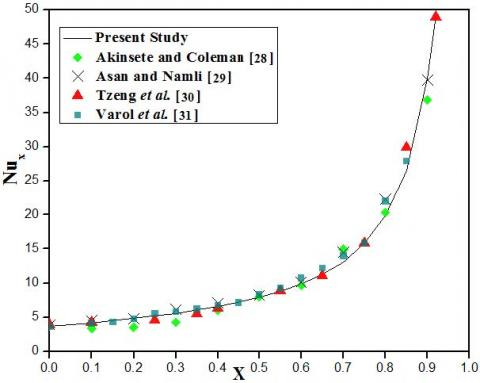Figure 3. Assessment of the present work with other published data for triangular cavity at Ra = 2772

4.2 Effects of hot wall aspect ratio

This section delineates, shown in Fig. 4, the behavior of streamlines (left) and isotherms (right) caused by aspect ratio of the caterpillar shape hot wall at fixed Rayleigh number; Ra = 5 x 105. It can be seen from the figure that the three vortexes of streamline are formed inside the cavity for all aspect ratio of the hot wall. The bigger cell is shaped near the side cold wall and the contour is covered the utmost part of the cavity while the remaining cells however one at the right corner and another to the middle of the inclined wall. The smaller cells are rotated in clockwise while the larger one in counter clockwise direction. It is remarkable to note that the shapes of all three vortexes of the streamlines and the structure of the isotherms lines are seems relatively analogues with each other for all aspect ratio of the hot wall. But, the maximum flow rate of the fluid decreases with the increase of σ. It describes that the flow motion of the fluid is getting reluctant because of the curvilinear shape yet it enhances the intensity of rate of heat transfer as the fluid particles are contacted with the hot part for longer duration.

## 4.pngFigure 4. Variation in streamlines (left) and isotherms (right) for caterpillar hot wall at a) σ = 0, b) σ = 0.05, c) σ = 0.1, d) σ = 0.15 for Ra = 5 x 105

Fig. 5 displays the local Nusselt number with non-dimensional distance X and Fig. 6 shows the average Nusselt number with aspect ratio of the hot wall and both are presented for the same Rayleigh number 5x 105 for better comprehension. The local Nusselt number, in Fig. 5, is presented the assessment of heat transfer for triangular and curvilinear hot wall with the smooth hot wall at σ = 0.05. In case of smooth hot wall, the local Nusselt number values are mounted constantly up to X = 0.7, after that it increases abruptly. It means the high heat transfer rate is occurred merely at the right corner. However when the smooth wall replaced from triangular zig-zag and caterpillar curve, the local Nusselt number escalated at every crest because the additional numbers of fluid particles come in contact with the hot wall due to the irregularity in the shape. In triangular heating, the fluid particles are moved in a zig-zag way which made the circulation flow sluggish while this situation is not occurred in curvilinear heating. Also, the curvilinear curve shape increase the surface area compare to other. That’s why, the higher value of local Nusselt number is found to be more for curvilinear hot wall in contrast of triangular and smooth hot walls.

## 5.jpgFigure 5. Variation in local Nusselt number with distance X for different shapes of the hot wall at σ = 0.05 and Ra = 5x105

The global variation of the heat transfer can also be analyzed from the average Nusselt number plot which shown in Fig. 6. In case of triangular heating the average Nusselt number is increased linearly with the aspect ratio however it is enhanced nonlinearly for curvilinear wall.

## 6.jpg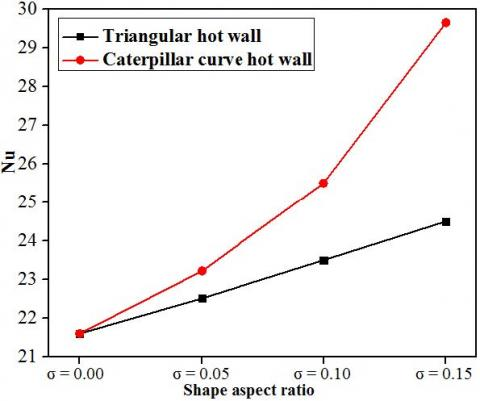Figure 6. Average Nusselt number variation with aspect ratios of the hot wall for different profiles of the hot wall at Ra = 5x105

The average Nusselt number, Nu, of triangular zig-zag wall for σ = 0.05, σ = 0.1 and σ = 0.15 is 4.21%, 8.79% and 13.43% respectively higher than plane wall. On the other hand, the average Nusselt number, Nu of curvilinear wall for σ = 0.05, σ = 0.1 and σ = 0.15 is 7.5%, 18.1% and 37.3 % respectively higher than smooth heating. Nevertheless, if we compare the average Nusselt number between triangular and curvilinear heating, the average Nusselt number of curvilinear heating at σ = 0.05, σ = 0.1 and σ = 0.15  is 3.5%, 8.51% and 21% respectively higher than triangular heating. Hence, it is clear that the caterpillar curve wall is better that the triangular and smooth wall.

4.3 Effect of Rayleigh number

## 7.pngFigure 7. Streamlines (left column) and Isotherms (right column) for C-curve hot wall with n = 0.1 at a) Ra = 1x105 b) Ra = 5x105 c) Ra = 1x106 d) Ra = 5x106 and e) Ra = 1x107

This part enlightens the streamlines and isotherms as shown in Fig. 7 for triangular zig-zag hot wall at σ = 0.1 for different Rayleigh numbers. It is known that the temperature gradient of the fluid in increased with the increment in Rayleigh number. A strong buoyancy force is being buildup inside the cavity with the increment in the temperature gradient and hence the flow pattern of the fluid is changed significantly. From streamlines, it is observed that the two vortexes at Ra = 1x105, three at Ra = 5x105 and 1x106 and four vortexes are formed at Ra = 5x106 and 1x107. At low Rayleigh number, the smaller temperature gradient is unable to setup a strong buoyancy force and thus the heat carrying capacity by the fluid particles is less. Two asymmetric circulating cells are formed such that the larger one on the left side whereas the another on right side at lower Rayleigh number. The left cell rotates in anticlockwise and the right cell, on the contrary, in clockwise direction. The central cell of eddies are circular which indicates about the sluggishness of the fluid movement. The rotational cells split into three cells and later on in to four cells at higher Rayleigh number. This detachment of the circulation eddies are signifies the increment in the movement of the flow with the increase of Rayleigh number. The motion of the flow can also be comprehend through stream function values which shown under each figures in Fig. 7.  The –ve sign of the stream function shows the rotation of fluid in clockwise and +ve sign in anticlockwise direction. The magnitude of the mean stream function is found, Ψmax = 15.25 at Ra = 1x105, Ψmax = 27.50 at Ra = 5x105, Ψmax = 39.18 at Ra = 1x106, Ψmax = 72.50 at Ra = 5x106 and Ψmax = 90.52 at Ra = 1x107.

## 8.pngFigure 8. Variation in local Nusselt number with distance X for triangular hot wall (left side) and caterpillar curve hot wall (right side) at different aspect ratio for  increasing Rayleigh number

Fig. 8 illustrates the influence of triangular heating (left column) and caterpillar curve shape heating (right column) on local Nusselt number for different Rayleigh number. The fluid particles get heat energy input from the base hot wall, get heat up and move because of the buoyancy driving force. The cold fluids pushed the hot fluids towards the right side and acquired their places as the density of hot fluid is less than the cold fluid. This phenomenon continues, sets fluids circulating throughout the whole cavity and transports the heat from hot wall to cold walls. In case of smooth hot wall, the local Nusselt number distributed gradually up to X = 0.6 after that the large variation in the local Nusselt number is found. It happens in the right triangular cavity because of the constriction between hot and cold walls. At the right corner of the cavity, conduction is more dominated over convection since some fluid particles got trapped due to the compactness along with this the heat transfer is convection dominant beside the corner of the cavity. Thus, it can be said that both mode of the heat transfer is actively possessed to extract heat from the right side of the cavity. In contrast, the local Nusselt number is mounted at every peak point of the triangular and curvilinear walls as the more numbers of fluid particles participated in the heat transport process due to their shapes and it also increases with the increment of their aspect ratio. The higher value of the local Nusselt number is calculated at σ = 0.15 for both cases. It is remarkable to note that the local Nusselt number value for curvilinear heating is approximately more than double then the triangular heating for each aspect ratio. In triangular heating, the fluid particles moves up and down because of its corrugated structure and generate a back flow which decrease the flow rate of the fluid however this phenomena is not occurred in case of caterpillar curvilinear hot wall. So, the heat transfer rate is higher in curvilinear heating than the triangular heating.

## 9.jpg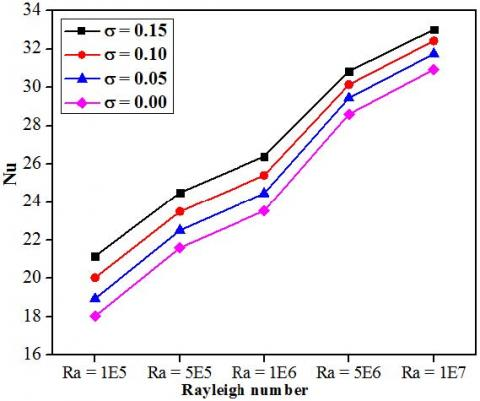Figure 9. Variation in average Nusselt number with Rayleigh number for triangular hot wall of different aspect ratio

It is also noticed that the heat transfer significantly enhances with the increasing Rayleigh number which can be seen in the Fig. 9 and 10. At low Rayleigh number the heat energy input to fluid from the base hot wall is less due to which a weak buoyancy force is occurred and hence the heat transfer is not full of convection dominant. As the Rayleigh number increases, the temperature gradient of the fluid is also increases. The increment in the temperature gradient increases the heat energy input to the fluid which sets the strong buoyancy force inside the cavity. This augmentation in the buoyancy force establishes a strong regime of convection heat transfer inside the cavity which leads to the enhancement of the average Nusselt number. For triangular heating, at Ra = 1x105, the average Nusselt number, Nu, is 5.04%, 11.1% and 17.3% for σ = 0.05, σ = 0.1 and σ = 0.15 respectively more than smooth heating. Furthermore, at Ra = 1x107, the average Nusselt number, Nu, is 2.65%, 4.85% and 6.76% for σ = 0.05, σ = 0.1 and σ = 0.15 respectively more than smooth heating. On the other hand, for curvilinear heating, at Ra = 1x105, the average Nusselt number, Nu, is 9.81%, 22.7% and 46.42% for σ = 0.05, σ = 0.1 and σ = 0.15 respectively more than smooth heating. Whereas, at Ra = 1x107, the average Nusselt number, Nu, is 5.07%, 11.4% and 23.18% for σ = 0.05, σ = 0.1 and σ = 0.15 respectively more than smooth heating. Nevertheless, if we compare the average Nusselt number between triangular and curvilinear heating, the average Nusselt number for curvilinear heating, Nu, is 24.82%, 21.02%, 19.32%, 17.06% and 15.38% at Ra = 1x105, 5x105, 1x106, 5x106 and 1x107 respectively higher than triangular heating.  Hence, from the data it can be conclude that both the shapes of the hot wall is performed better than the smooth hot wall at lower Rayleigh number than compare to the higher values of Rayleigh number. Even though if we compare between them, caterpillar curve hot wall is performed better than the corrugated shape hot wall at lower Rayleigh number.

## 10.jpg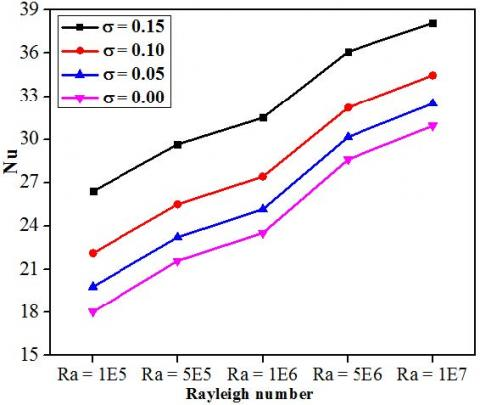Figure 10. Variation in average Nusselt number with Rayleigh number for caterpillar curve hot wall of different aspect ratio

5. Conclusion

Table 2. Average Nusselt number for different shapes of hot wall

 Ra = 1E5 Ra = 5E5 Ra = 1E6 Ra = 5E6 Ra = 1E7 SW 18.03 21.60 23.53 28.60 30.93 TZ σ = 0.05 18.94 22.51 24.46 29.45 31.75 σ = 0.10 20.03 23.50 25.40 30.14 32.43 σ = 0.15 21.15 24.50 26.40 30.84 33.02 CS σ = 0.05 19.80 23.22 25.17 30.16 32.50 σ = 0.10 22.12 25.51 27.42 32.20 34.46 σ = 0.15 26.40 29.65 31.50 36.10 38.10

The numerical study of natural convection heat transfer has been analyzed in a right angle triangular cavity. The cavity has been heated isothermally from bottom through smooth, triangular shape and caterpillar shape curve wall and cooled from vertical and inclined walls. The aspect ratio of the triangular and curvilinear hot wall is changed from σ = 0 to 0.15. The sole purpose of the present investigation was to examine the influence of the different shapes of hot wall with different heights on heat transfer rate. The problem has been examined for Rayleigh number (Ra = 105 – 107). From the analysis it has been found that the placing the corrugated shape wall and caterpillar shape curve wall instead of smooth wall affects the fluid rotation and enhances the local and average Nusselt number. Both, triangular and curvilinear wall can be used as base hot wall based on the design of system component and rate of cooling. The heat transfer rate increases with the increase of aspect ratio and found higher at σ = 0.15 for both triangular and curvilinear hot walls. Also, the heat transfer rate is enhanced with the increase in Rayleigh number for all the cases. But, the caterpillar curve shape hot wall bestows the higher heat transfer rate than the others which is shown in table 2. The admirable percentage increment in the heat transfer rate is found at low Rayleigh number compare to other Rayleigh number.

Acknowledgment

The authors acknowledge the precious and productive comments suggested by the reviewers which have been great utilized to improve the quality of this manuscript.

Nomenclature

g             gravitational acceleration

H            cavity height

L            cavity width y

s             hypotenuse of the cavity

h            height of triangular zig-zag and caterpillar curve shapes

w           shape width

p           pressure

P             dimensionless pressure

u, v        velocity components

U, V       dimensionless velocity components

x, y       co-ordinates system

X, Y     dimensionless co-ordinates system

T           temperature

Th          hot wall temperature

Tc          cold wall temperature

Pr          prandtl number

Ra         Rayleigh number

Nux        local Nusselt number

Nu         average nusselt number

Greek symbols

α           thermal diffusivity

β           coefficient of thermal expansion

θ         dimensionless temperature

k         conduction coefficient

ρ         density

σ         curve aspect ratio (h/w)

µ         dynamic viscosity

υ          kinematic viscosity

ψ         stream function

Ψ         dimensionless stream function

Subscripts

h           hot wall

c           cold wall

References

 Pesso T., Piva S. (2009). Laminar natural convection in a square cavity: low prandtls numbers amd large density differences, Int. J. Heat Mass Transfer, Vol. 52, pp. 1036–1043. DOI: 10.1016/j.ijheatmasstransfer.2008.07.005

 Deng Q.H. (2008). Fluid flow and heat transfer characteristics of natural convection in square cavities due to discrete source–sink pairs, Int. J. Heat Mass Transfer, Vol. 51, pp. 5949–5957. DOI: 10.1016/j.ijheatmasstransfer.2008.04.062

 Wu W., Ewing D., Ching C.Y. (2006). The effect of the top and bottom wall temperatures on the laminar natural convection in an air-filled square cavity, Int. J. Heat Mass Transfer, Vol. 49, pp. 1999–2008. DOI: 10.1016/j.ijheatmasstransfer.2005.11.027

 Bocu Z., Altac Z. (2011). Laminar natural convection heat transfer and air flow in three-dimensional rectangular enclosures with pin arrays attached to hot wall, Applied Thermal Engg, Vol. 31, pp. 3189–3195. DOI: 10.1016/j.applthermaleng.2011.05.045

 Ramakrishn D., Basak T., Roy S., Momoniat E. (2014). Analysis of thermal efficiency via analysis of heat flow and entropy generation during natural convection within porous trapezoidal cavities, Int. J. Heat Mass Transfer, Vol. 77, pp. 98–113. DOI: 10.1016/j.ijheatmasstransfer.2014.04.002

 Mahmoodi M. (2011). Numerical simulation of free convection of a nanofluid in L-shaped cavities, Int. J. Thermal Science, Vol. 50, pp. 1731–1740. DOI: 10.1016/j.ijthermalsci.2011.04.009

 Basak T., Aravind G., Roy S. (2009). Visualization of heat flow due to natural convection within triangular cavities using bejan’s heatline concept, Int. J. Heat Mass Transfer, Vol. 52, pp. 2824-2833. DOI: 10.1016/j.ijheatmasstransfer.2008.10.034

 Nasr K.B., Chouikh R., Kerkeni C., Guizani A. (2006). Numerical study of the natural convection in cavity heated from the lower corner and cooled from the ceiling, Applied Thermal Engineering, Vol. 26, pp. 772–775. DOI: 10.1016/j.applthermaleng.2005.09.011

 Hasanuzzaman M.,  Rahman M.M., Öztop H.F., Rahim N.A., Saidur R. (2012). Effects of Lewis number on heat and mass transfer in a triangular cavity, Int. Comm. Heat Mass Transfer, Vol. 39, pp. 1213–1219. DOI: 10.1016/j.icheatmasstransfer.2012.07.002

 Kent E.F. (2009). Numerical analysis of laminar natural convection in isosceles triangular enclosures for cold base and hot inclined walls, Mechanics Research Communications, Vol. 36, pp. 497-508. DOI: 10.1016/j.mechrescom.2008.11.002

 Kaluri R.S., Anandalakshmi R., Basak T. (2010). Bejan’s heatline analysis of natural convection in right-angled triangular enclosures: effects of aspect-ratio and thermal boundary conditions, Int. J. Thermal Sciences, Vol. 49, pp. 1576-1592. DOI: 10.1016/j.ijthermalsci.2010.04.022

 Varol Y., Oztop H.F., Mobedi M., Pop I. (2007). Visualization of natural convection heat transport using heatline method in porous non-isothermally heated triangular cavity, Int. J. Heat Mass Transfer, Vol. 51, pp. 5040-5051. DOI: 10.1016/j.ijheatmasstransfer.2008.04.023

 Mahmoudi A., Mejri I., Omri A. (2016). Study of natural convection in a square cavity filled with nanofluid and subjected to a magnetic field, Int. J. Heat Technology, Vol. 34, pp. 73-79. DOI: 10.18280/ijht.340111

 Triveni M.K., Sen D., Panua R.S. (2014). Laminar natural convection for thermally active partial side walls in a right-angled triangular cavity, Arab. J. Sci. Engg, Vol. 39, pp. 9025-9038. DOI: 10.1007/s13369-014-1418-7

 Triveni M.K., Sen D., Panua R.S. (2015). Natural convection in a partially heated triangular cavity with different configurations of cold walls, Arab. J. Sci. Engg, Vol. 40, pp. 3285-3297. DOI: 10.1007/s13369-015-1778-7

 Bouabdallah S., Ghernaout B., Teggar M., Benchatti A., Benarab F. (2016). Onset of natural convection and transition laminar-oscillatory convection flow in rayleigh-bénard configuration, Int. J. Heat Technology, Vol. 34, pp. 73-79. DOI: 10.18280/ijht.340122

 Dalal A., Das M.K. (2005). Laminar natural convection in an inclined complicated cavity with spatially variable wall temperature, Int. J. Heat Mass Transfer, Vol. 48, pp. 2986–3007. DOI: 10.1016/j.ijheatmasstransfer.2004.07.051

 Bhardwaj S., Dalal A., Pati S. (2015). Influence of wavy wall and non-uniform heating on natural convection heat transfer and entropy generation inside porous complex enclosure, Energy, Vol. 79, pp. 467–481. DOI: 10.1016/j.energy.2014.11.036

 Rahman M.M., Saidur R., Mekhilef S., Uddin M.B., Ahsan A. (2013). Double-diffusive buoyancy induced flow in a triangular cavity with corrugated bottom wall: effects of geometrical parameters, Int. Comm. Heat Mass Transfer, Vol. 45, pp. 64–74. DOI: 10.1016/j.icheatmasstransfer.2013.04.002

 Hatami M., Safari H. (2016). Effect of inside heated cylinder on the natural convection heat transfer of nanofluids in a wavy-wall enclosure, Int. J. Heat Mass Transfer, Vol. 103, pp. 1053-1057. DOI: 10.1016/j.ijheatmasstransfer.2016.08.029

 Cho C.C. (2016). Influence of magnetic field on natural convection and entropy generation in Cu–water nanofluid-filled cavity with wavy surfaces, Int. J. Heat Mass Transfer, Vol. 101, pp. 637-647. DOI: 10.1016/j.ijheatmasstransfer.2016.05.044

 Sheremet M.A., Revnic C., Pop I. (2017). Free convection in a porous wavy cavity filled with a nanofluid using Buongiorno’s mathematical model with thermal dispersion effect, Applied Mathematics Computation, Vol. 299, pp. 1-15. DOI: 10.1016/j.amc.2016.11.032

 Shirvan K.M., Ellahi R., Mamourian M., Moghiman M. (2016). Effects of wavy surface characteristics on natural convection heat transfer in a cosine corrugated square cavity filled with nanofluid, Int. J. Heat Mass Transfer, Vol. 107, pp. 1110-1118. DOI: 10.1016/j.ijheatmasstransfer.2016.11.022

 Sheremet M.A., Pop I., Ishak A. (2017). Time-dependent natural convection of micropolar fluid in a wavy triangular cavity, Int. J. Heat Mass Transfer, Vol. 105, pp. 610-622.  DOI: 10.1016/j.ijheatmasstransfer.2016.09.044

 Yapici K., Obut S. (2015). Laminar mixed-convection heat transfer in a lid-driven cavity with modified heated wall, Heat Transfer Engineering, Vol. 36, pp. 303–314. DOI: 10.1080/01457632.2014.916160

 Fluent User’s Guide, Release 6.3.26. (2005), Fluent Incorporated.

 Yesiloz G., Aydin O. (2013). Laminar natural convection in right-angled triangular enclosures heated and cooled on adjacent walls, Int. J. Heat Mass Transfer, Vol. 60, pp. 365-374. DOI: 10.1016/j.ijheatmasstransfer.2013.01.009

 Akinsete V.A., Coleman T.A. (1982). Heat transfer by steady laminar free convection in triangular enclosures, Int. J. Heat Mass Transfer, Vol. 25, pp. 991 – 998. DOI: 10.1016/0017-9310(82)90074-6

 Asan H., Namli L. (2000). Laminar natural convection in a pitched roof of triangular cross-section: summer day boundary conditions, Energy and Buildings, Vol. 33, pp. 69–73. DOI: 10.1016/S0378-7788(00)00066-9

 Tzeng S.C., Liou J.H., Jou R.Y. (2005). Numerical simulation-aided parametric analysis of natural convection in a roof of triangular enclosures, Heat Transfer Engineering, Vol. 26, pp. 69–79. DOI: 10.1080/01457630591003899

 Varol Y., Oztop H.F., Yilmaz T. (2007). Natural convection in triangular enclosures with protruding isothermal heater, Int. J. Heat Mass Transfer, Vol. 50, pp. 2451-2462. DOI: 10.1016/j.ijheatmasstransfer.2006.12.027